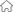您现在的位置：首页 > 新闻中心 > 水质公告
2023年9月水质公告

 `水质指标` `国家标准（GB5749-2006）` `出厂水检测结果` `限 值` `单 位` `柳林水厂` `南湖水厂` `郢都水厂` `总大肠菌群` `不得检出` `MPN/100mL` `未检出` `未检出` `未检出` `耐热大肠菌群` `不得检出` `MPN/100mL` `未检出` `未检出` `未检出` `菌落总数` `100` `CFU/mL` `未检出` `未检出` `未检出` `色度` `15` `/` `<5` `<5` `<5` `浑浊度` `1` `NTU` `0.32` `0.28` `0.29` `臭和味` `无异臭、异味` `/` `无` `无` `无` `肉眼可见物` `无` `/` `无` `无` `无` `耗氧量` `3` `mg/L` `1.20` `0.96` `1.02` `余氯` `≥0.3` `mg/L` `0.66` `0.60` `0.60` `检测员` `瞿丽霞、刘晓莉 ` `贺小力 、罗敏` ` 马常娟、将旻静`
```说明：以上数据为荆州市各水厂出厂水监测结果本月平均值。

```